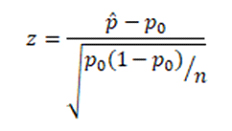# One proportion test

Statistics are very complicated, hence people depends on the data analysis and thus performs tests. There are multiple parameters that you can test. Like, for z-test (paired-sample-test), it is a test for the mean. The one proportion test is commonly done to confirm or debunk claims. Test for the population proportion is denoted as p. The only thing you need to do is take a random sample of components and use one proportion test to determine that the actual proportion backs up the claim. For example, you want to know the proportion of males within a total population of adults. Here test of proportion helps you assess whether the sample represents the true proportion from the entire population or not. There are a few things that needed to be defined first before conducting the proportion test.

## There are two ways to perform a test of proportion;-

### Critical value Approach

1. State the null hypothesis H0 and the alternative hypothesis HA.
2. Calculate the test statistic:1. where p0 is the null hypothesized proportion i.e. when H0:p=p0
2. Determine the critical region.
3. And lastly, determine if the test statistic falls in the critical region. As, If it does fall in it, reject the null hypothesis. If it does not, do not reject the null hypothesis.

### p-value Approach

1. State the null hypothesis H0 and the alternative hypothesis HA.
2. Set the level of significance α
3. Calculate the test statistic:
4. Calculate the p-value.
5. Decide by checking whether to reject the null hypothesis by comparing p-value to α. Also, If the p-value < α then reject H0; otherwise do not reject.

## What is a one-proportion Z-test?

This test is performed to compare an observed proportion to a theoretical one when there are only two categories. Based on the sample size, normal approximation or binomial enumeration is done. If the sample size is large, the normal approximation provides an accurate result. If the sample size is less, then binomial enumeration gives the correct results. The binomial enumeration and normal approximation can be approximated when both mean (np) and variance( n(1-p)) values are greater than 10.

## Compute one proportion z-test in R

R functions: binom.test() & prop.test()

• The R functions binom.test() and prop.test() can be used to perform one-proportion test:
1. binom.test(): compute exact binomial test. Recommended when the sample size is small
2. prop.test(): can be used when the sample size is large ( N > 30). It uses a normal approximation to binomial

The syntax of the two functions are the same. The simplified format is as follow:

binom.test(x, n, p = 0.5, alternative = "two.sided")

prop.test(x, n, p = NULL, alternative = "two.sided",

correct = TRUE)

1. x: the number of successes
2. n: the total number of trials
3. p: the probability to test against.
4. correct: a logical indicating whether Yates’ continuity correction should be applied where possible.

You can also send us an email   or call us directly on, (+44) 20 3287 0255 and will get in touch with you shortly

I had to submit a big report within a few days. I thought it was impossible to do so. But experts from SPSS Tutor Saved the day.

Paul

had complex research about a medical condition. It was difficult to finish the tasks. But thanks to the SPSS tutor, they helped me to finish tasks on time and made it look easy.

Emily

I had to write an assignment for an IT topic. It required research on new technology. Finding information about it was difficult because it is new. But the SPSS tutor team helped me with it and today I got the highest grades in the class.

Andrew

They helped me with my statistics homework. The team is friendly, the service is nice and I liked how they kept asking me suggestions. I loved their service and would recommend it to others.

Kimberly

Get Instant Quote